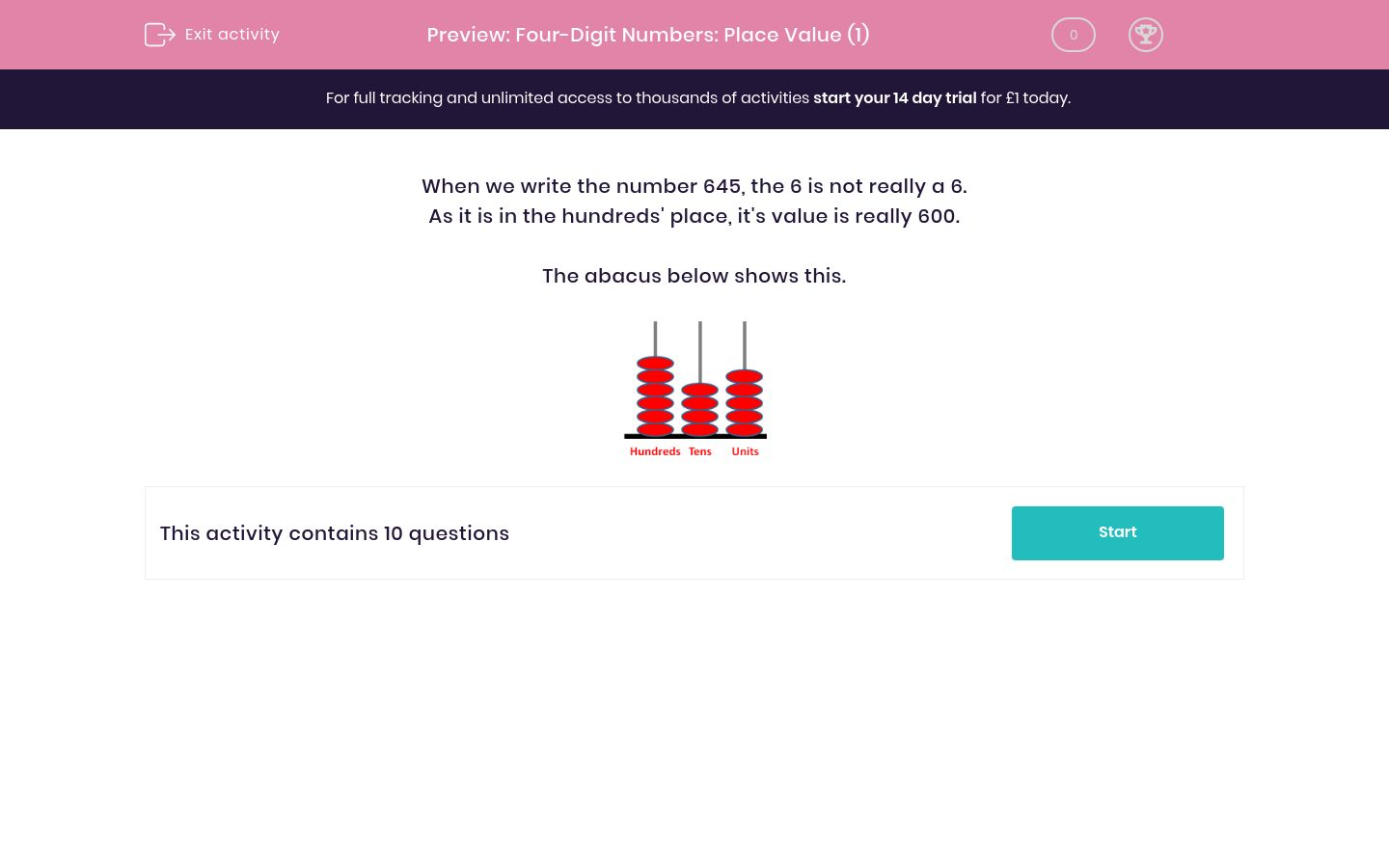# Four-Digit Numbers: Place Value (1)

In this worksheet, students give the 'value' of a digit in a number.Key stage:  KS 2

Curriculum topic:   Maths and Numerical Reasoning

Curriculum subtopic:   Place Value

Difficulty level:### QUESTION 1 of 10

When we write the number 645, the 6 is not really a 6.

As it is in the hundreds' place, it's value is really 600.

The abacus below shows this.What is the real value of the digit 5 in the number 257 ?

5

50

500

What is the real value of the digit 5 in the number 527 ?

5

50

500

What is the real value of the digit 5 in the number 275 ?

5

50

500

What is the real value of the digit 2 in the number 275 ?

2

20

200

What is the real value of the digit 2 in the number 327 ?

2

20

200

What is the real value of the digit 2 in the number 372 ?

2

20

200

What is the real value of the digit 8 in the number 278 ?

8

80

800

What is the real value of the digit 8 in the number 800 ?

8

80

800

What is the real value of the digit 8 in the number 801 ?

8

80

800

What is the real value of the digit 8 in the number 891 ?

8

80

800

• Question 1

What is the real value of the digit 5 in the number 257 ?

50
• Question 2

What is the real value of the digit 5 in the number 527 ?

500
• Question 3

What is the real value of the digit 5 in the number 275 ?

5
• Question 4

What is the real value of the digit 2 in the number 275 ?

200
• Question 5

What is the real value of the digit 2 in the number 327 ?

20
• Question 6

What is the real value of the digit 2 in the number 372 ?

2
• Question 7

What is the real value of the digit 8 in the number 278 ?

8
• Question 8

What is the real value of the digit 8 in the number 800 ?

800
• Question 9

What is the real value of the digit 8 in the number 801 ?

800
• Question 10

What is the real value of the digit 8 in the number 891 ?

800
---- OR ----

Sign up for a £1 trial so you can track and measure your child's progress on this activity.

### What is EdPlace?

We're your National Curriculum aligned online education content provider helping each child succeed in English, maths and science from year 1 to GCSE. With an EdPlace account you’ll be able to track and measure progress, helping each child achieve their best. We build confidence and attainment by personalising each child’s learning at a level that suits them.

Get started